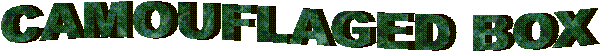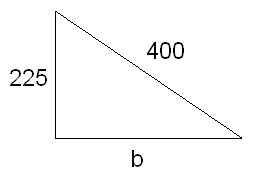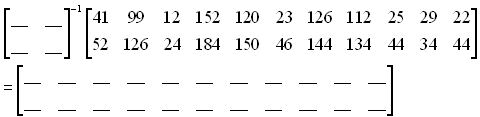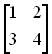Mathman 14 Cedar Creek Letterbox Dani Kieselhorst, Heather Aderman, Sam Seider, and Amanda WilkensTerrain: Grassy, can be muddy or even flooded at times Difficulty: Depends on math ability Placed by: The Dragon (Mathman) and his Honors Advanced Algebra students of 2004-05 Location: Cedar Creek Park in Cedarburg, WI County: Ozaukee, WI Materials needed: Calculator, Compass, Writing Utensil, Personal Stamp, Inking PensStudents and parents of students should read my introduction to letterboxing before seeking the boxes.

These clues rely on the use of matrices to encode/decode clues.  Go to this page to learn how to do this on a free graphics calculator simulator or to do it by hand.

Be sure to read through all the clues before looking for the boxes so you know what is expected of you.

Clues:

1. If 1=N, 2=E, 3=W, and 4=S, then solve the following function for the positive solution of x to determine which parking lot to park in (this is only the general direction, not exact):

f(x) = 3x2 + 12x – 96

Take Hwy 60 west of Grafton to "5 Corners".  Take Covered Bridge Rd north from "5 corners" about a mile to the park. Park in the lot indicted by the solution above.

2. Once the car is parked in the correct parking lot, proceed to count the number of garbage cans on the perimeter of  this parking lot.

Number of garbage cans (h) = ______

Multiply h by the value of x in the following equation’s vertex point:

y = 2x2 – 28x + 90

hx = ______

Walk southwest hx number of paces from the light post in the northern area of the parking lot.

3. You should now be at an inconspicuous landmark. To figure out which direction to head in, use your compass. To figure out the degrees you need to find on the compass, find the value of b in this right triangle (round to the nearest whole number):4. Proceed in the direction of your new-found bearing for about 12π (12pi) paces. Upon reaching your destination, check to see if the smaller decorative polygons on its sides satisfy the Pythagorean Theorem. If they don’t, continue on. If they do, then start doing the clues over starting from where your car is parked.  (Don't worry, the clues will wait for you to catch up.)

Continue until you reach a path. To make sure you should follow this potentially misleading path, solve the following equation:

y = x2 – 309x + 21800

The solution of lesser value will equal the number of paces to walk. The solution of greater value will equal what direction to travel when N=200, E=150, S=100, and W=50.

5. Hopefully you are now where you are supposed to be. If you are, you are highly encouraged to count the number of rocks between the nearest light post and the V-shaped tree nearby.

Number of rocks (r) = ______

Find r3 and use this 4-digit number as the decoder in the following matrix:Note:  As an example, the decoder 1234 would be placed in the matrix as:6. After following the decoded directions, turn south when you meet an intersection. Beware of motorized mechanical devices! Observe the speed limit sign which did not face you before you looked at it.

Record the MPH (s) = ______

Find s2 and use this 4-digit number as the decoder for the following matrix:7. Make your way to the object named in the above matrix. Count all the diamonds on the east side of the object.

Number of diamonds (d) = _____

Take d and multiply by p and then multiply by zero.

(Hopefully, you read ahead and realized that counting the diamonds serves no purpose except to strengthen your counting skills.)

8. Find a sign with some historical information near said object. Read to discover when this object was built and retired.

Built (b) = _____

Retired (r) = _____

B and R are inversely related (B = K/R).  Find the constant of variation (k).   Divide the constant of variation by pp  and round to the nearest whole number.

Whole number (K) = _____

Then find X if A varies directly as A using the constant of variation K from above. (A = KX)
Have A = 706622 multiplied by the larger of the two numbers on the sign which have to do with dimensions.

X = _______

9. Then, identify this type of function:  f(x) = [[x]] or f(x) = INT(x)

This type of function will tell you where you should stand close by to start counting paces to your final destination. Count off X paces at a bearing of 359 degrees.

BEFORE RETRIEVING THE BOX, BE SURE NO ONE IS AROUND TO SEE YOU TAKE THE BOX OUT!  DO NOT BRING ATTENTION TO YOURSELF OR THE HIDING LOCATION OF THE BOX!

From there, walk carefully on the stone ledge on the outside.  There you will find a prickly bush that has been cut back to its stump in front of some large rocks.  Directly behind the stump are three asphalt rocks.  Remove them to expose the hiding location of the box.  Please replace the rock well so the box cannot be seen.

Before you set out read the waiver of responsibility and disclaimer.

LbNA Home